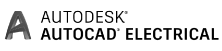## Technical Info

Small pumping stationsPumping stationsSSS pumping stationsFigure PDFFigure DWGControl cabinetsFigure PDFFigure DWGCertificateFigure PDFFigure DWGCertificateFigure PDFFigure DWGCertificateFigure PDFFigure DWGCertificateFigure PDFFigure DWGCertificateUnderground tanksFigure PDFFigure DWGCertificateAboveground tanksFigure PDFFigure DWGFigure PDFFigure DWGFigure PDFFigure DWGFigure PDFFigure DWGFigure PDFFigure DWGFigure PDFFigure DWGFigure PDFFigure DWGSeptic tanksManholes for potable waterFigure PDFFigure DWGFigure PDFFigure DWGFigure PDFFigure DWGFigure PDFFigure DWGWastewater and stormwater pipesFigure PDFFigure DWGFigure PDFFigure DWGCertificateCable well OptoCertificateWelding rodCertificateCertificatePE, PP, PVDF pipes and fittingsFigure PDFFigure DWGInstallation GuideCatalogue## DOCUMENTATION

For design we always use licensed software. For production drawings we use the most popular CAD system in the world – SolidWorks 3D CAD. For strength calculations we use SolidWorks Simulation and to prepare the control cabinet schemes and layout we use AutoCAD Electrical software.